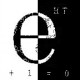# 直上九宵的指数方程

1.est
Sep 16 2009

> 因为最低下的x的指数是他本身

这个错了吧？

•Sep 20 2009

这里没有什么问题啊 最低下的x的指数和他本身形式是一模一样的

2.严酷的魔王
Sep 16 2009

又记得是在哪里看过的- –

3.Sep 16 2009

“把这个数顶上的√2用更大的数2来替代，则整个表达式坍缩为2，所以结果是不可能大于2的。”

这样的描述是有问题的，顶上根本就没有一个“√2”可以被替换掉，因为顶上还是“√2的√2循环次”，也就是仍然是x，如果假设这个x=4,你替换成2,显然是把这个x变小了，所以变成2并不为怪。
也就是说光这样并不能说明x不可能大于2。

•Sep 20 2009

我那么说确实有些不严谨哎 不过是最简单的理解方式…

4.sss正和
Sep 17 2009

函数y=x^x^x^…当x在(0, e^1/e)时上界为e，但当x=e^(1/e)时y突然变成无穷大了。
因为x=e^(1/e)时，x^x^x^…=(e^(1/e))^(e^(1/e))^(e^(1/e))^(e^(1/e))^…=e^e^(1+e^(1+e^(1+e^………..)))
令e^(1+e^(1+e^(1+e^………..)))=y，自相似代换得e^(1+y)=y，无解，即x^x^x^…为无穷大。

所以y=x^x^x^..=4>=e无解，强行做自相似代换y=x^y也只得到增根。

•sss正和
Sep 18 2009

上帖犯了一个低级错误，更正如下：
x^x^x^…=c的自相似代换是x^c=c，即x=c^(1/c)，在c=e时x取得极值e^(1/e)。
于是x>e^(1/e)时x^x^x^…的值为无穷大。因为x=e^(1/e)+ε=e^e^(δ-1)时，
x^x^x^…=(e^e^(δ-1))^(e^e^(δ-1))^(e^e^(δ-1))^…=e^e^(δ-1+e^(δ-1+e^(δ-1+e^………..)))
令e^(δ-1+e^(δ-1+e^(δ-1+e^………..)))=z，自相似代换得e^(δ-1+z)=z，当δ>0时无解（或者说解为无穷大），即x>e^(1/e)时x^x^x^…为无穷大。

函数y=x^x^x^…当x在(0, e^1/e]时上界为e，但当x>e^(1/e)时y突然变成无穷大了。
所以x^x^x^..=4>e无解，强行做自相似代换也只得到增根。

•Sep 20 2009

对 你这个解释严谨的正解..只不过这样表述比较繁琐…

5.Sep 17 2009

x怎么会大于1呢？
f(x)>f(x)^x，在x>1时

•sss正和
Sep 18 2009

你应当把x^x^x^…看成x,x^x,x^x^x,…这样一个数例的极限。然后自己用excel试算一下，x1,c>1时，可以有x^c<=c这才是关键，保证数列通项可以有上限。

•sss正和
Sep 18 2009

x1应是x>1

6.Sep 18 2009

为什么会坍缩为2呢？

•Sep 20 2009

因为√2的2次方还是2..不断的算下来最后还是2

7.星光居士
Sep 19 2009

总觉得第二个方程的解有问题。

•Sep 20 2009

就是有问题..它应该是无解的…

8.zyzyzhangyuan
Sep 21 2009

既然同一个式子可以有不同的值，岂不是说这个式子不收敛？如果不收敛，讨论这个问题就没有意义。

一个例子是，y=1/x,当x=0的时候就没有意义，因为不收敛，当然它也可以有两个值……

这种无穷项的叠代的方法都是逻辑不严谨的方法，都应该是有前提的，都是要求收敛甚至有的要求绝对收敛的。

√2上有1个√2是1.6几，两个就是2，4个就是4啊。这样会不断的变大的，而且速度很快，肯定趋于无穷大的。

•Sep 21 2009

你用计算器稍微验证一下就可以知道 这个确实是收敛的 极限确实是2

9.pia
Sep 21 2009

这个悖论产生的原因源自于做法……
我们这里设a1=x
a(n+1)=x^an

而这种做法只用了递推公式
并未用到初始条件

例如上面的悖论，若初始条件2<a1<4就会得到极限为4，其余当大于4时，则会去想无穷大

所以只是初始条件的问题…….

•Sep 21 2009

按照那个式子 a1应该是√2的
这个问题有意思的地方在于 如果是让我单独解第二个方程 我肯定解出来x=√2就觉得解对了完事儿了…

•pia
Sep 22 2009

所以很无奈的
第一个式子即使算出来解也不能算对

必须代回去重新证明……

而且证明貌似也挺麻烦的……

10.jcvb
Nov 11 2009

用程序算一下 答案是2
第2个方程好像无解吧。。

11.kights
Dec 1 2009

可以把第一个式子（那么多个√2）设为n
因为式子里有无数个√2
那么在式子下面再加一个√2也不会影响原式结果
所以有(√2)^n=n
显然 n=2

•kights
Dec 1 2009

不知道这样是否合理？

12.创业计划书
Dec 2 2009

换域名啦。来看看

13.choniong
Apr 16 2010

对于方程x^x^x^…=m似乎只有当m在[1/e,e]上时才存在唯一的x使方程成立，而当m在(0,1/e)上时用原帖的方法解得x后，x^x^x^…的值会根据x的个数的奇偶性在2个不同的极限间跳动。
不知道我做的对不对？

14.napolen
Aug 19 2010

为何我就没有学过这种求解方法呢，看了半天还是没懂。。

15.Nov 21 2010

“因为最低下的x的指数是他本身”, 什么叫”他本身”? 会歧义好不好, “方程左项”不会说是吧?

“把这个数顶上的√2用更大的数2来替代”, 注意, 无穷, 无穷是什么懂不懂? “顶上”永远是方程左项, 先假设了方程左项不会超过2, 然后推出方程左项不会超过2, 真有追求…

用初等代数玩级数, 这是玩给初中生看的吧, 现在高中都学级数了

16.17.